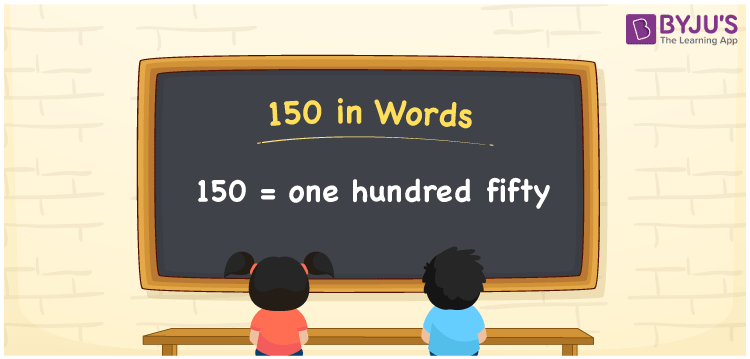# 150 in words

150 in words is written as One Hundred and Fifty. 150 represents the count or value. The article on Counting Numbers can give you an idea about count or counting. The number 150 is used in expressions that relate to money, days, distance, length, weight and so on. Let us consider an example for 150. “The distance between Bangalore and Mysore is around One Hundred and Fifty kilometres.” ”I need to write an essay that has exactly 150 words”. “I purchased a tiny Refrigerator with a capacity of 150L”.

 150 in words One Hundred and Fifty One Hundred and Fifty in Numbers 150

## 150 in English Words## How to Write 150 in Words?

We can convert 150 to words using a place value chart. The number 150 has 3 digits, so let’s make a chart that shows the place value up to 3 digits.

 Hundreds Tens Ones 1 5 0

Thus, we can write the expanded form as:

1 × Hundred + 5 × Ten + 0 × One

= 1 × 100 + 5 × 10 + 0 × 1

= 150

= One Hundred and Fifty.

150 is the natural number that is succeeded by 149 and preceded by 151.

150 in words – One Hundred and Fifty.

Is 150 an odd number? – No.

Is 150 an even number? – Yes.

Is 150 a perfect square number? – No.

Is 150 a perfect cube number? – No.

Is 150 a prime number? – No.

Is 150 a composite number? – Yes.

## Solved Example

1. Write the number 150 in expanded form

Solution: 1 × 100 + 5 × 10 + 0 × 1

We can write 150 = 100 + 50 + 0

= 1 × 100 + 5 × 10 + 0 × 1

## Frequently Asked Questions (FAQs) on 150 in Words

Q1

### How to write the number 150 in words?

150 in words is written as One Hundred and Fifty.
Q2

### State True or False. 150 is divisible by 3?

True. 150 is divisible by 3.
Q3

### Is 150 a perfect square number?

No. 150 is not a perfect square number.